# Addition on a Number Line

The addition on a number line helps us to find the sum of any two real numbers easily, given the numbers are small.

While adding two numbers on a number line, initially, we locate the first number and then move to the right or left according to the value of the second number.

Let us add two integers 4 + 5 on a number line.

Since both are positive integers, we move towards the right starting from the first number. The second number decides the number of steps to move.

As we know, 4 + 5 = 9; let us perform this addition operation step by step on a number line.

Step 1: A number line with numbers from 0 to 10 is drawn. As the first number, 4, is positive, it is marked by moving 4 units to the right of 0.

Step 2: The second number, 5, is also positive, so we move 5 more steps to the right of 4.

Thus we find the result 9 on the number line.

Calculate 8 + 7 using a number line.

Solution: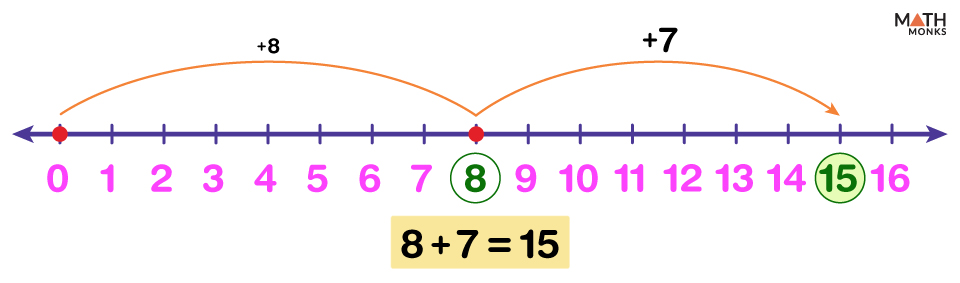Here a number line with numbers from 0 to 16 is drawn. The first number, 8, is plotted to the right of 0. Then, moving 7 steps to the right of 8, we find the answer 8 + 7 = 15

## With a Positive and a Negative Number

Just like adding two positive numbers, we can also add a positive and a negative number using a number line. There can be two possible cases:

Case 1: First Number is Positive, and the Second Number is Negative

Suppose we add 7 + (-3).

Step 1: The first number, 7 being positive, is marked by moving 7 units to the right of 0.

Step 2: As the second number (-3) is negative, in this case, the direction of movement will be towards the left, starting from the first number. So we take 3 steps to the left starting from the first number, i.e., 7.

The result 7 + (-3) = 4 is on the number line.

Case 2: First Number is Negative, and the Second Number is Positive

Let us add (-12) + 7 and find the result on a number line.

Step 1: The first number (-12), being negative, is placed by moving 12 steps to the left of 0.

Step 2: Next, we move 7 steps to the right of the first number, i.e., (-12), as the number to be added (i.e., 7) is positive.

The result (-12) + 7 = (-5) is obtained on the number line.

Calculate (-0.4) + 0.2 on a number line.

Solution:As the first number (-0.4) lies between 0 and (-1), the area from 0 to (-1) is divided into 10 equal parts, and (-0.4) is located by moving 4 steps to the left of 0.
Then the second number, 0.2 being positive, we move 2 steps to the right of (-0.4).
Thus we get the result (-0.4) + 0.2 = (-0.2) on the number line.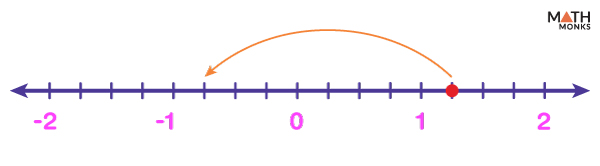Which addition equation matches the following number line?
a) ${1\dfrac{1}{4}}$ + ${\left( -\dfrac{3}{4}\right)}$ =  ${\dfrac{2}{4}}$
b) ${1\dfrac{2}{4}}$ + (-2) =  ${ -\dfrac{2}{4}}$
c) c) ${1\dfrac{2}{4}}$ + ${\left( -\dfrac{3}{4}\right)}$ =  ${ \dfrac{3}{4}}$
d) ${1\dfrac{1}{4}}$ + (-2) =  ${ -\dfrac{3}{4}}$

Solution: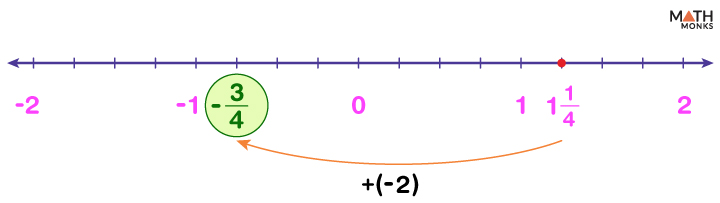The given number line has 4 equal parts between each consecutive integer. Looking at the fractional numbers between each pair of integers, we see that the arrow starts from ${1\dfrac{1}{4}}$ and ends to the left of ${1\dfrac{1}{4}}$, at ${\left( -\dfrac{3}{4}\right)}$. Also, there are a total of 8 equal jumps of ${\dfrac{1}{4}}$ each between them.
Thus the number to be subtracted from of ${1\dfrac{1}{4}}$ is 8 × ${\dfrac{1}{4}}$ = 2
In other words, the number to be added to ${1\dfrac{1}{4}}$ is 8 × ${\left( -\dfrac{1}{4}\right)}$ = (-2) and the result is ${ -\dfrac{3}{4}}$.
Hence the correct addition equation is option d) ${1\dfrac{1}{4}}$ + (-2) =  ${ -\dfrac{3}{4}}$.

## With Two Negative Numbers

While adding two negative numbers, the direction of movement will be toward the left.

Suppose we want to find the result of (-9) + (-1).

Step 1: As the first number (-9) is negative, it is marked 9 units to the left of 0.

Step 2: The second number (-1) also being negative, 1 more step are taken to the left starting from (-9)

Thus, we find the result (-9) + (-1) = -10 on the number line.

Find (-3) + (-6) using a number line.

Solution: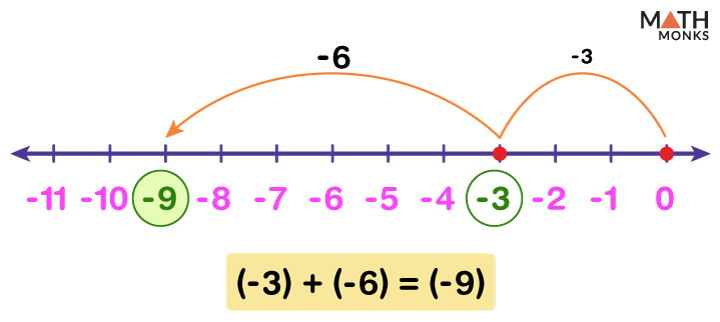First, (-3) is located to the left of 0, and 6 more steps are taken to the left starting from (-3).
The sum (-3) + (-6) = (-9) is obtained on the number line.

We can also add multi-digit numbers, such as 2-digit and 3-digit numbers, on a number line.

Let us add 68 and 53 using an open number line.

Step 1: Since the first number 68 is positive, it is plotted to the right of 0 on an open number line.

Step 2: We decompose the second number, i.e., 53, and count the 10s and 1s.

Step 3: Here, as 53 = (5 × 10) + (3 × 1), 5 longer jumps and 3 shorter jumps are taken to count forward by 10s five times and 1s three times to the right of 68. After every jump, each number (78, 88,… 120, 121) is marked on the number line.

We get the result 121 on the number line.

Add 763 and 256 using a number line.

Solution: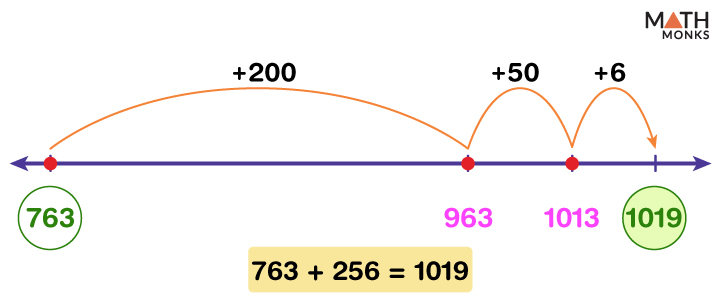The first number, 763, being positive, is marked to the right of 0 on an open number line.
As the second number 256 = (2 × 100) + (5 × 10) + (6 × 1), so it has two 100s, five 10s and six 1s.
1 longer jump to count forward by 200, 1 moderate jump to count forward by 50, and 1 shorter jump to count forward by 6 are taken to the right of 763. All the numbers (963, 1013, 1019) are marked after each jump.
Thus, we find the result 1019 on the number line.